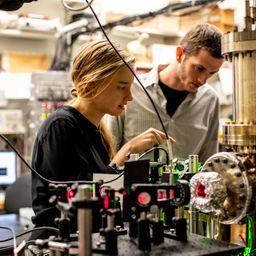## a. 1.90 \mathrm{eV}b. 0.216 \mathrm{V}

Quantum Physics

Atomic Physics

### Discussion

You must be signed in to discuss.
##### Top Physics 103 Educators##### Christina K.

Rutgers, The State University of New Jersey##### Andy C.

University of Michigan - Ann Arbor##### Aspen F.

University of Sheffield### Video Transcript

okay. And this problem, we're told we have two light sources and we want to find the work function for a particular metal surface. And so we have wavelength one. It's 5 46.1 Nanami interests. We can call that Lambda One is 5 46 0.1 times 10 to the minus nine meters and and then V one is equal to 10.376 So you want a sequel to 0.376 rules And, um, you reduces the photo current to zero Great. And then So we want to get based on this. What is the work function? Um, for the metal. So let's use this formula that applies generally to the for the photoelectric effect that the stopping voltage Time Z is equal to the energy of the photons. That's issue over Lambo minus five. So you get that fei is equal to HC over lambda minus e times this stopping voltage. So I'm gonna, um, plug all of this information into a calculator so I could do each time, see, provided by from 5 46.1 nano meters and then from that what I want to do a subtract um, one free e. So 1.6, if you slipped into the minus 19 and multiplied by the voltage, that's 0.376 And so I got the work function, and then I have that in units of, um, duels. And so what I want to do is provided by, uh, we're quite a six times 10 to the minus 19 to get it ready. Notes of evey. So I got the work function. Is one plane to 89? Um, TV. So that's the answer for a and then, huh? We want to get the stopping potential. Um, so So that's you took for a So the stopping potential. Now, we're gonna take this same equation right here and solve it for V. And so you got that. B is equal to one over e times, HC over Lambda minus. Um Oh, And then we have this new lamb. This all call this Lambda too. So, uh, yes, it's time to To minus by and then I'll go ahead and plug in that land. It too, is equal to 5 87.5 you know, meters. And then if I put all of that into a calculator C five, maybe seven points. I new meters, and I want to subtract the work function. All right, Let's see. Oh, yeah, I know. I want to check the work section functions. That's 1.73 Where? 1.9. Excuse me. And let me just make sure I have everything in the proper unit. So this isn't Jules. This is Jules. Great. And so I got. So I got for the potential 0.2 to 1 bolds. So let's pause and make sure this makes sense. So I have, um, higher, higher, an energy light, and then I need a bigger potential than if I have lower energy. Like Great. Yeah, that makes sense to me. Nearly star passes that check. Um, I'm just gonna double checks with my math preparations. 6.1.University of Washington

#### Topics

Quantum Physics

Atomic Physics

##### Top Physics 103 Educators##### Christina K.

Rutgers, The State University of New Jersey##### Andy C.

University of Michigan - Ann Arbor##### Aspen F.

University of Sheffield